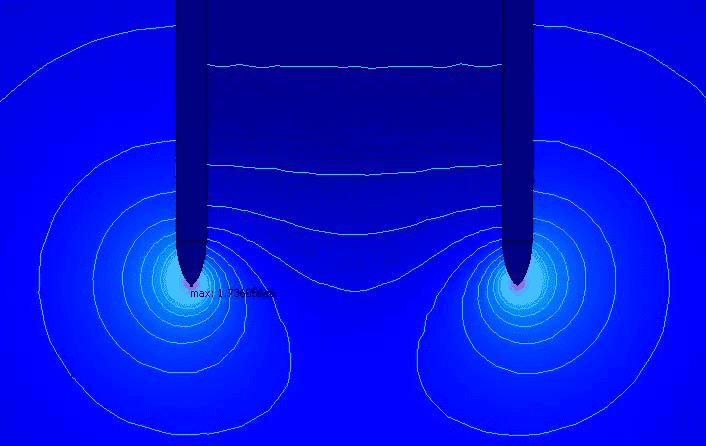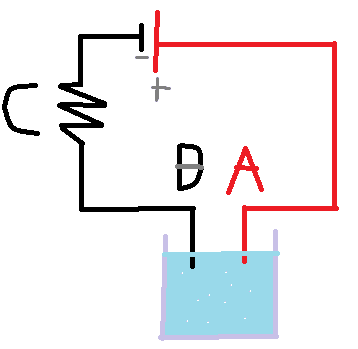# Salt water and potential difference

Tryhard314
Imagine a container of salt water at 0V (Relative to ground),Now you've put in it 2 electrodes,one at +500V (Electrode A), The other at +250V(Electrode b), Normally positive ions should go to the negative electrode , and Negative ions should go to the positive electrode , But in our example the salt water is at 0V So you would have to do positive work to go to those electrodes. But because a negative charge do a negative work to reach a higher potential, they will be attracted to both electrodes, But there would be a higher attraction for the +500V than the 250+,

My Idea is this: With more and more negative ions leaving the salt water to get dissolved in the electrodes, Salt water potential will rise until it's >250V because if salt water potential raises more than +250V positive ions will start being forced to go to the 250V electrode because it's at a lower potential, and no more negative ions will go to the +250V because they would need to do positive work to go from salt water to that electrode.

Further more I think salt water's potential will reach exactly 375V, because at this value the potential difference between Electrode A (500V) and Salt water(375V) = 125V, and the potential difference between Salt water(375V) and electrode B (250V) = 125V
So the number of positive ions that go the Electrode B is equal to the number of negative ions that go to the electrode A

jartsa
What does "potential of salt water" mean?

Can we model this situation as a resistor whose two ends are connected to two potentials, or as a conductor whose two ends are connected to two potentials?

In the first case potential somewhere between the electrodes is between the potentials of the electrodes.

In the second case there is a huge explosion, I mean something must break in the second case.

Last edited:
•Tryhard314
Mentor
Thread is in Moderation temporarily for review...

Last edited:
Mentor
Further more I think salt water's potential will reach exactly 375V, because at this value the potential difference between Electrode A (500V) and Salt water(375V) = 125V, and the potential difference between Salt water(375V) and electrode B (250V) = 125V
So the number of positive ions that go the Electrode B is equal to the number of negative ions that go to the electrode A
It depends on how conductive the salt water solution is. If it's very conductive, it will short out your voltage source. If it's not very conductive, you will end up with electric field lines defining the potentials and the voltages inside the "grounded" equipotential cylinder and between the electrodes. Think of extending this simulation plot to the case where there is a surrounding equipotential cylinder...

https://encrypted-tbn0.gstatic.com/...-aUKtQMbl-CC5sUK4mmSTfmgRuWwl_nuwBh7DKp5LLw&sLast edited:
•Tryhard314
Mentor
I think salt water's potential will reach exactly 375V, because at this value the potential difference between Electrode A (500V) and Salt water(375V) = 125V, and the potential difference between Salt water(375V) and electrode B (250V) = 125V
There is a current through the salt water so by ohms law the voltage will vary within the water itself. Near electrode B it will be near 250 V and near electrode A it will be near 500 V.

•Tryhard314
Homework Helper
But in our example the salt water is at 0V
You can't keep it there with ony two electrodes at +250 and +500 V

Tryhard314
There is a current through the salt water so by ohms law the voltage will vary within the water itself. Near electrode B it will be near 250 V and near electrode A it will be near 500 V.
After writing for 1 hour,I realized that in my first post i worked for the average value of potential inside the salt water,and you just described how the potential gradient is inside the salt water because there can't be a direct drop from +500 to 375V ,I leave you this paint image of my circuit if someone want to use it someday And Thanks !•Nugatory, berkeman and Dale
••